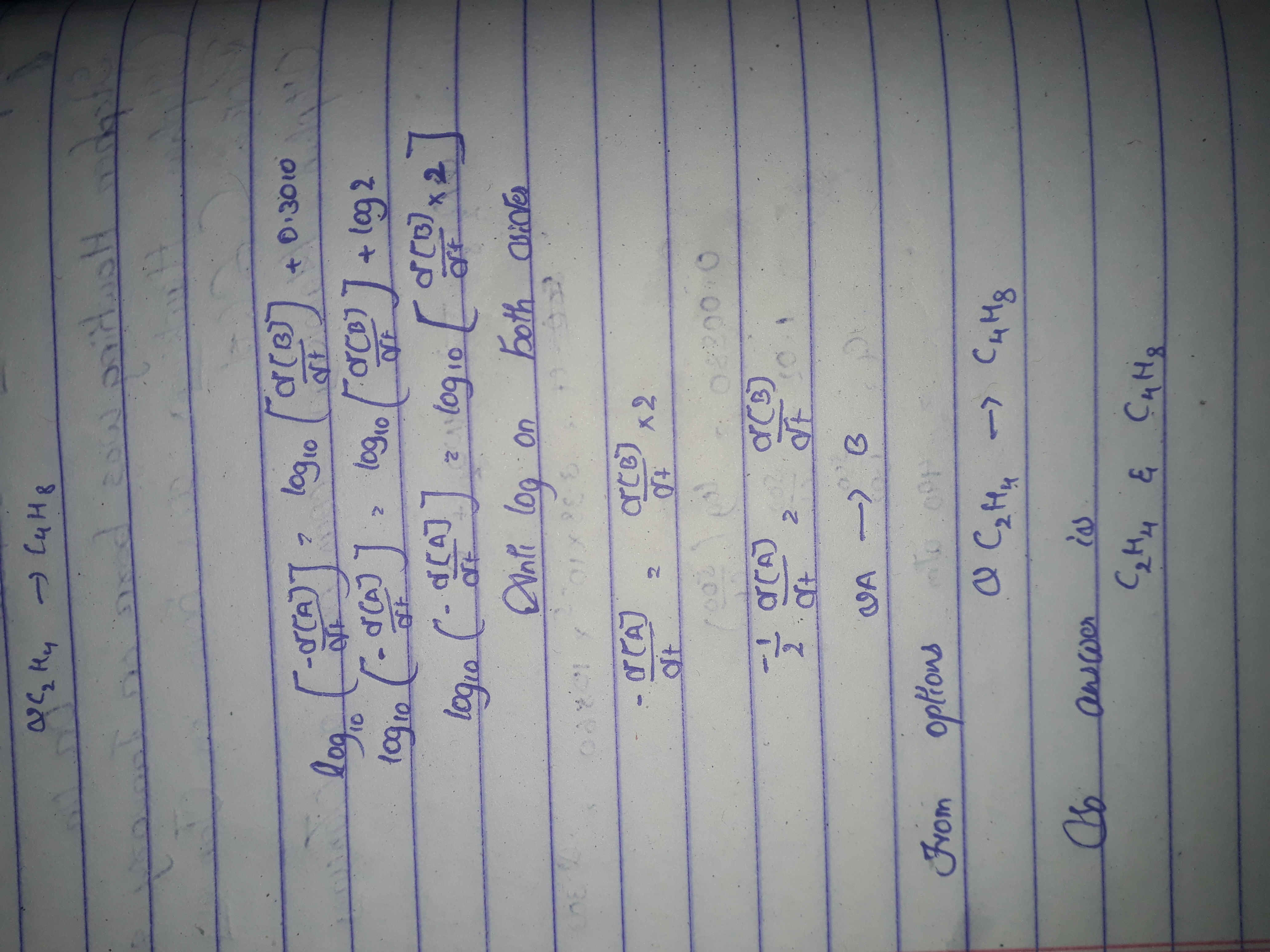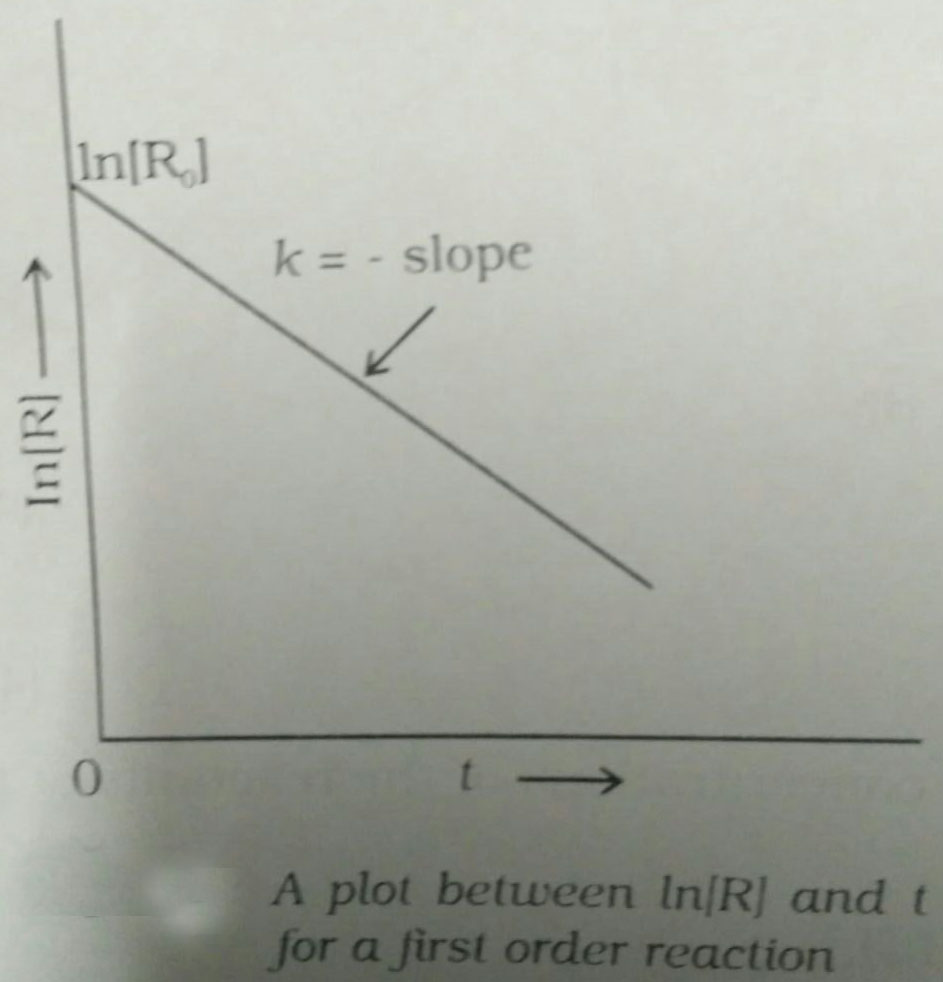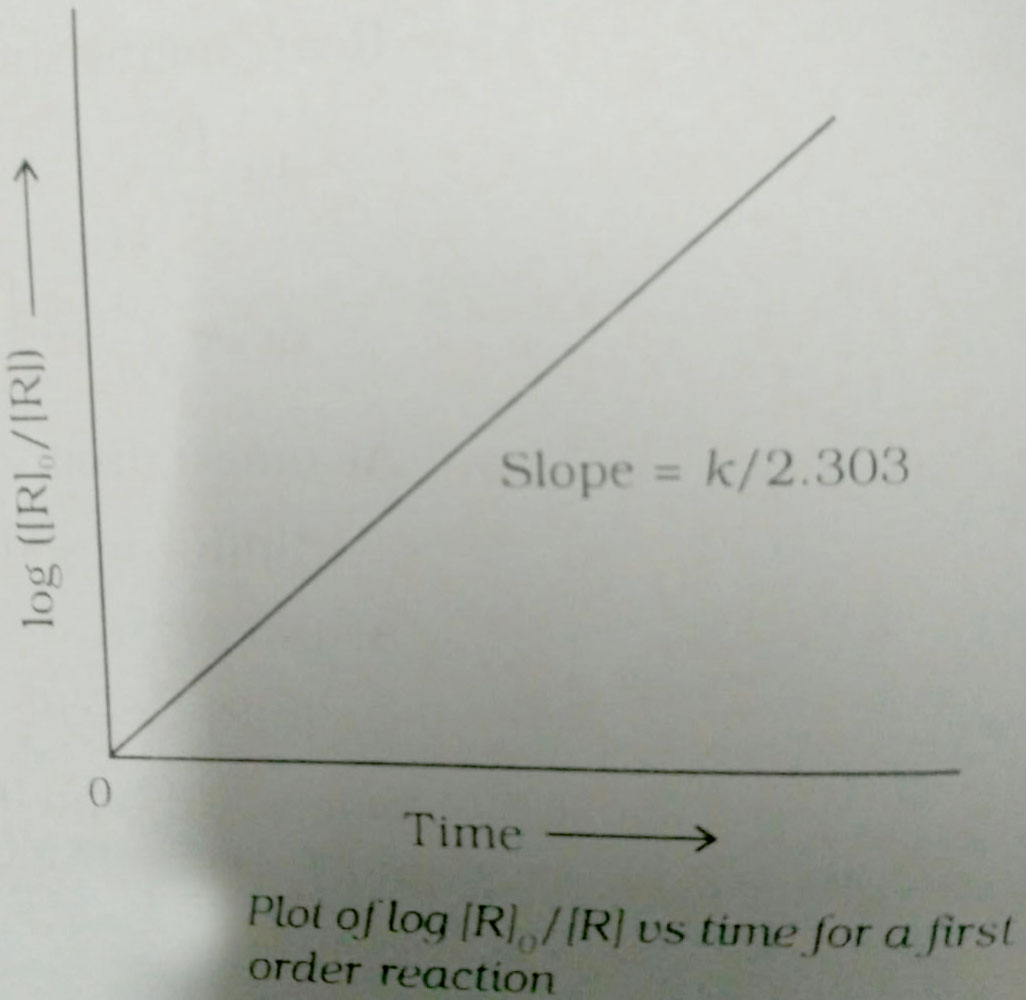Q

# Solve it, - Chemical kinetics - JEE Main

In the following reaction;

'A' and 'B' respectively can be :

• Option 1)

and

• Option 2)

and

• Option 3)

and

• Option 4)

n-Butane and Iso-butane

ViewsFirst Order Reaction -

The rate of the reaction is proportional to the first power of the concentration of the reactant.- wherein

Formula:

R            P

a                 0

a-x             x

[differentiate rate law]

Unit of

&

&

Option 1)

and

Option 2)

and

Option 3)

and

Option 4)

n-Butane and Iso-butane

Exams
Articles
Questions